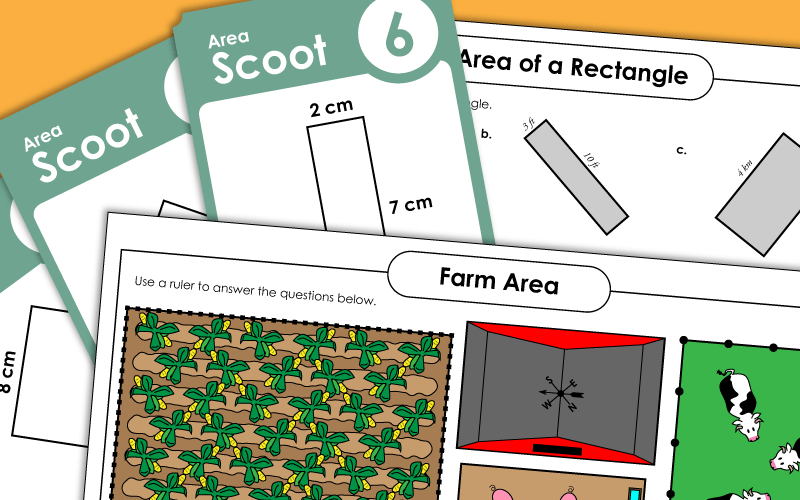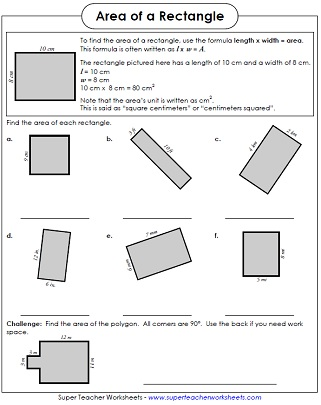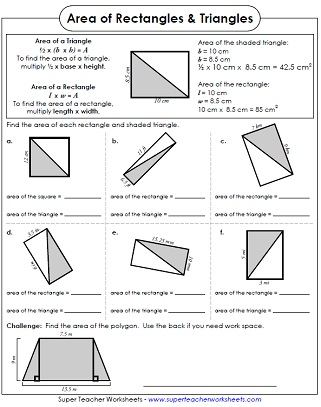# Area of Rectangles and Squares

Below you'll find several printable geometry worksheets on finding the areas of rectangles. Students will practice using the formula "area equals length times width."## Basic

Calculate the areas of the given rectangles by multiplying the width by the length.  Requires only basic multiplication skills.
In part 1, students find the area of each rectangle. In part 2, they are given the area and must find the length of a side.
Find the area of each rectangle by multiplying the length by the width.
Write the area of each rectangle in the picture. Which one has the largest area? Which two have the same area?
Draw a quadrilateral on the grid that has 20 square units. On the next grid, draw a square that has an area of 4 units. Then draw a square with an area that is 9 times greater.
This file contains 30 task cards. Print them out and use them for learning centers, peer study groups, classroom scavenger hunts, or small group activities.
In this game, students move from desk to desk, around the classroom, and calculate the area of rectangles.
In this whole-class chain reaction game, students must find the area of a shape on a card, and be ready to announce it when his card is called.

## Intermediate

Students must multiply to find the areas of the rectangles shown.  Requires knowledge of 2-digit multiplication.

Students must use their multiplication skills to find the areas of the rectangles shown.  Requires knowledge of 2-digit multiplication, multiplying decimals, and multiplying fractions.
Use your ruler to measure the pigs' pen, the corn field, and the cows' pasture.  Multiply to find the area of each.
Calculate the area of each triangle and rectangle picture.  This activity teaches the relationship between the areas of right triangles and rectangles.  Requires knowledge of 2-digit decimal multiplication.

Area of Irregular Shapes (Rectilinear Figures)

On these worksheets you'll find the areas of irregular shapes. The shapes are made up of 2 or more rectangles (rectilinear figures).

More Area Worksheets

Find the areas of triangles, circles, trapezoids, and more.

Area of Triangles

These worksheets focus specifically on the finding areas of triangles.

Perimeter Worksheets

Students must find the perimeter, or distance around a shapes, on each of these worksheets.

Surface Area

Calculate the surface area of rectangular prisms and other three-dimensional shapes.My Account
Site Information